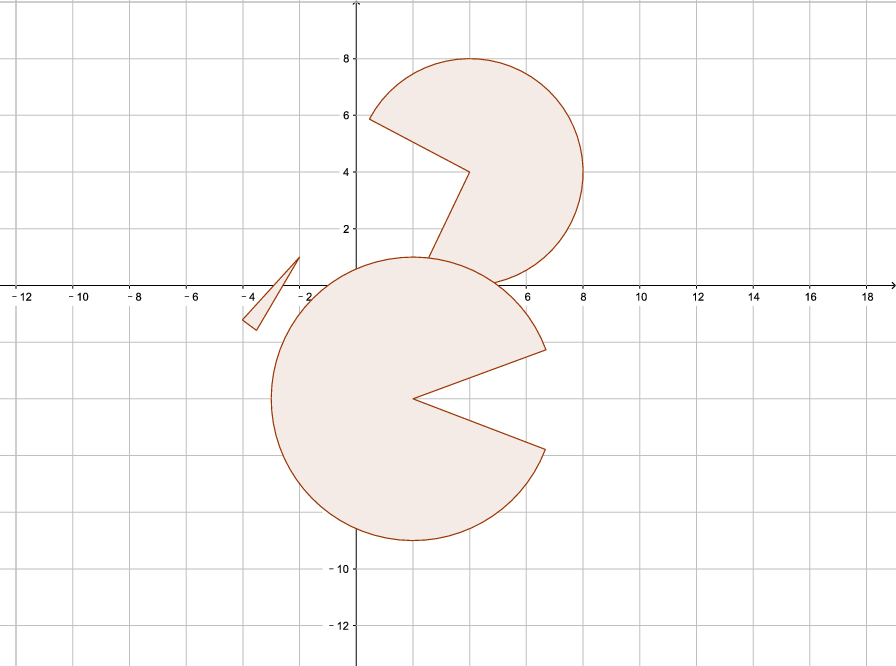시간 제한메모리 제한제출정답맞힌 사람정답 비율
2 초 256 MB43266.667%

## 문제

Bobo has drawn $n$ circular sectors on the plane. He would like to know the area of the union of all the circular sectors.

## 입력

The input contains zero or more test cases, and is terminated by end-of-file. For each test case:

The first line contains an integer $n$, the number of circular sectors ($1 \leq n \leq 500$).

Each of the next $n$ lines contains five numbers $x_i$, $y_i$, $r_i$, $s_i$ and $\theta_i$ ($-100 \le x_i, y_i \le 100$, $1 \le r_i \le 100$, $0 \le s_i \le 6$, $0.1 \le \theta_i \le 6$). Here, ($x_i, y_i$) is the coordinate of the circle center, $r_i$ is the radius of the circle, $s_i$ is the starting angle in radians (counter-clockwise from the positive direction of the $x$ axis) and $\theta_i$ is the central angle in radians (this means that the sector arc goes from angle $s_i$ to angle $s_i + \theta_i$ where the angle is measured counter-clockwise from the positive direction of the $x$ axis). Also, $x_i$, $y_i$ and $r_i$ are integers, and $s_i$ and $\theta_i$ are real numbers with exactly $3$ digits after the decimal point.

It is guaranteed that the sum of $n$ does not exceed $500$.

## 출력

For each test case, output a real number denoting the answer. Your answer will be considered correct if its relative or absolute error doesn't exceed $10^{-6}$.

## 예제 입력 1

2
-3 -5 5 0.705 0.217
-5 1 4 3.070 4.136
1
-4 -4 1 0.485 2.260
3
4 4 4 4.266 4.673
2 -4 5 0.353 5.565
-2 1 3 3.974 0.207


## 예제 출력 1

35.80050000000000700000
1.12999999999999940000
106.44493143870359000000


## 힌트

The image below shows the third test case.